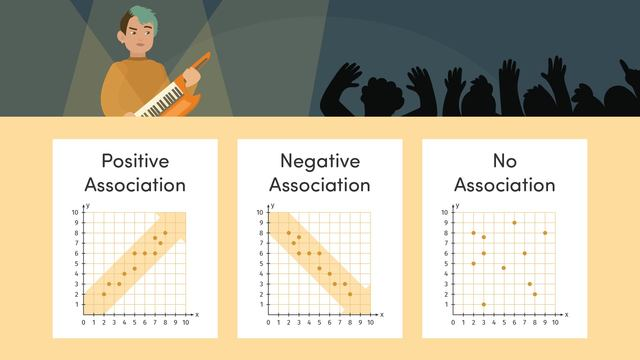# Describing Patterns in Scatter Plots

Content Describing Patterns in Scatter PlotsRating

Be the first to give a rating!
The authorsTeam Digital

## Scatter Plot Introduction

A scatter plot is a type of graph that shows the relationship, or association, between two different sets of data, which we call bivariate data. By looking at a scatter plot, we can see patterns such as how the data points cluster, if there are any unusual points (outliers), and whether the relationship is positive, negative, linear, or nonlinear.

## Understanding Scatter Plot

A scatter plot helps us see if there's a link between two variables. For example, it can show if one thing increases, does the other thing tend to increase too? This is a positive association. Or if one decreases as the other increases, that's a negative association. When the points don't show any obvious pattern, we say there's no association. If the points make a straight line, that's a linear association. If they form a curve, it's a nonlinear association.

## Scatter Plot Example

Example Problem:

Create a scatter plot for the following data that shows the number of hours students studied and their test scores:

Hours Studied Test Score
1 50
2 60
3 65
4 70
5 80

Solution:

1. Draw a graph with two axes: horizontal for hours studied and vertical for test scores.
2. Mark each pair of values as a point on the graph.
3. Observe the pattern that the points make.

## Scatter Plot - Guided Practice

How would you plot the point for someone who studied 2 hours and got a score of 60?
What does it mean if most of the points on the scatter plot seem to be rising from left to right?

## Scatter Plot - Your Turn!

Example Problem:

Plot the following data on a scatter plot:

Hours Studied Test Score
1 80
2 78
3 75
4 70
5 65
Construct the scatter plot.
Describe the association.

## Scatter Plot Summary

Scatter plots are a useful way to visualize the relationship between two variables. By plotting bivariate data and analyzing the pattern of points, we can determine if there is an association—whether it's positive, negative, linear, or nonlinear. Understanding scatter plots can help us make predictions and find trends in the data.